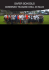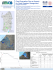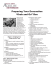# PRINT SAMPLE OF ENGLISH MANUSCRIPT

## Transcription

PRINT SAMPLE OF ENGLISH MANUSCRIPT
```Proceedings of Coastal Engineering, JSCE, Vol.3, 2012, November
NUMERICAL SIMULATION FOR EVACUATION
PROCESS AGAINST TSUNAMI DISASTER AT
TELUK BATIK IN MALAYSIA
BY MULTI-AGENT DEM MODEL
1Graduate School of Engineering, Department of Civil and Earth Resource Engineering, Kyoto University,
[email protected]
2 Department of Civil And Earth Resource Engineering, Kyoto University. [email protected]
3 Department of Civil And Earth Resource Engineering, Kyoto University. [email protected]
The development of good individual behavior model is of significance to simulate the crowd behavior. Our
research group has developed the Crowd Behavior Simulator for Disaster Evacuation (CBS-DE) based on the distinct
element method (DEM). This DEM-base model for a tracking individual behavior has contributed to investigate an
evacuation planning. Then the self-evasive action model has been introduced into the existing CBS-DE to simulate
more realistic crowd behavior. With using this simulator, the pedestrian behavior, such as an avoidance of collision and
an alignment between adjacent pedestrians are well reproduced in the contraflow. The contraflow may occurr in the
evacuation process due to an unexpected situation. Hence, in the present study, the self-evasive action model is
validated firstly by comparison with an observation, then numerical simulation for the evacuation process including
contraflow is performed at Teluk Batik Beach in Malaysia. The effect of the self-evasive action model is shown in the
context of the required time to complete evacuation.
Key Words: DEM, Self-evasive action Model, Evacuation analysis, Town planning.
comparison with CBS-DE simulation
with/without the self-evasive action model.
1. INTRODUCTION
The micro-simulator based on the distinct element
method (DEM) has been applied to evacuation
process by many researchers to simulate each
individual behavior including contact force (e.g.,
Park et al., 2004; Kiyono et al., 1996, 1998). And
each individual behavior model in the
micro-simulator have been improved to simulate
actual behavior. Our research group has developed
the Crowd Behavior Simulator (CBS) based on the
DEM. And problem for the evacuation process has
been investigated computationally by using the
Crowd Behavior Simulator for Disaster Evacuation
(CBS-DE) (e.g., Gotoh et al., 2004, 2008). In recent
year, the self-evasive model has been developed to
improve the individual behavior by Gotoh et al.
(2012). And the effect the self-evasive action model
on the simulation results for the contraflow at
crossing has been shown (Gotoh et al., 2012).
In this paper, the evacuation process against
tsunami disaster at Teluk Batik in Malaysia has been
carried out by using CBS-DE with self-evasive
action model. And the significance of the
description of individual behavior will be shown by
results
2. CROWED BEHAVIOR MODEL
(1) Fundamental equations
In this section, the CBS-DE with self-evasive action
model (Gotoh et al., 2012) is outlined. The
translational and rotational equations are employed
for simulate each individual behavior as follows:
mhi・
vi = Fmi + F ci + F ei
Ihi・
ωi = Ti
(1)
(2)
where vi = velocity of the person i, ωi = angular
velocity of the person i, "˙" = time-derivative, ・
vi =
acceleration of the person i, ・
ωi = angular
acceleration of the person i, Fmi = autonomous
driving force of the person i, F ci = interacting force
acting on the person i, F ei = self-evasive force
showing either collision avoidance action or an
alignment action, Ti = torque acting on the person i.
In the present study, each individual person is
simulated as a cylinder, thus, the mass and the
moment of inertia of the person i, mhi and Ihi
respectively can be written as:
- 56 -
psychological repulsive force
person i, fij = local physical repulsive force between
the persons i and j, fiW = local physical repulsive
force between the person i and the virtual wall
element W, fps_ij = local psychological repulsive
force between the persons i and j. These local
repulsive forces fij, fiW and fps_ij are given as follows:
physical repulsive force
psychological contact area (personal space)
physical contact area
element j
wall boundary
moving direction
Λ = 0.976m
 
 en
f com.  

element i
 
 t
+ e

Angle of vision
o
Θ = 120
element j
n
virtual wall element W
mhi   hi hi Bhi
d hi
4
; I hi   hi hi Bhi
d hi
32
(3)
(2) Interacting forces
Each individual person is controlled with the
perception domain mobile territory as illustrated in
Fig.1. Concerning to the physical repulsive force,
the following conditions are given.
ri  r j 
d hi  d hj
2
;
ri  rW 
d hi  dW
(4)
2
where ri = positional vector of the person i, rW =
positional vector of the virtual wall element W, d W =
diameter of the virtual wall element W. While, the
psychological repulsive force acts when two
adjacent persons i and j satisfy the following
condition:
The total force acting on the person i is
described as follows:
Fci = Fd(p/p)i + Fd(W/p)i + Fps_i
j (i )
j (i )
j (i )

1
Ti    (r j  ri )  fij   (rW  ri )  f iW  (8)
2  j ( i )
W

where Fd(p/p)i = physical repulsive force acting on
the person i, Fd(W/p)i = physical repulsive force
between the virtual wall element W and the person i,
Fps_i= psychological repulsive force acting on the
n
;
=f +ft
com.
t
f = et + dt
(10)
(3) Autonomous driving force
We assume that the isolated person is accelerated up
to the specific equilibrium velocity vlimit by the
autonomous driving force Fmi as follows:
Fmi = mi a
(14)
where a = acceleration vector. And the equilibrium
velocity is subjected to number density of human.
Therefore, the magnitude of the maximum
equilibrium velocity vmax is given as follows:
(6)
Fd(p/p)i = fij ; Fd(W/p)i =fiW ; Fps_i = fps_ij (7)
n
(9)
com.
n
where en and et = component of the repulsive force
due to the elastic springs, kn and kt = spring
constants in the normal and the tangential directions,
respectively, dn and dt = repulsive force due to the
viscosity dashpots, cn and ct = dashpot constants in
the normal and tangential directions, respectively,
f n and f t = total repulsive force in the normal and
tangential directions, respectively. The superscript
"pre" indicates the previous numerical time step,
rtarget represents the positional vector of the target
element. Subscript "com." indicates ij, iW and ps_ij,
and the corresponding the subscript "target" are the
person j and the virtual wall element W.
(5)
ri  r j  Λ
 k t r t t c t v n t 


et
d n 
Δrn =[rtarget –ri ]Δt·n ; Δrt =[rtarget –ri]Δt·t
d =| ri –rtarget|
n=(ri –rtarget)/d=(n1,n2) ; t=(-n2,n1)
Δvn = (vtarget – vi)·n ; Δvt = (vtarget – vi )·t
4
where σhi = density of the person i, Bhi= body height
of the person i, dhi = diameter of the person element
i, εhi = correction coefficient concerning the
volumetric difference between the cylinder element
and the actual person.
 k n r n n c n v nn 


en
d n 
f =e +d
Fig. 1: Illustration of perception domain of each human.
2
pre
pre
vmax = vlimit – γck
(15)
where γ is the proportional coefficient, vlimit =
specific equilibrium velocity in an isolated
walking condition, ck = number density of persons
inside the psychological perception area.
(4) Self-evasive force
In the present study, behavior of collision
avoidance and an alignment are described by a
- 57 -
Table 1: Setup procedure of model constants between
persons.
aaaa
dashpot
spring
physical repulsive
force, ij
kn
kn =1.26×104 N/m
psychological
repulsive force, ps ij
kn =
Ma
dh
2

kt
kn ×0.05
kn ×0.05
cn
2(Mkn)1/2
2(Mkn)1/2
ct
2(0.05Mkn)1/2
2(0.05Mkn)1/2
with the unit vector evi the avoidance force Fei acts
on pedestrian i in the right direction perpendicular
to the travelling direction of the pedestrian i.
Fig. 2: Perception domain for self-evasive action.
single model introducing the self-evasive force,
since both actions are closely related in each other.
The self-evasive force is estimated by referring the
predicted position of surrounding pedestrians after
Δtf seconds as shown in Fig. 2. Based on
observation by Tatabe et al. (1994), the turning
angle to avoid the static obstacle is Θa = ±15º and
radius of the perception domain for self-evasive
action is Λa = 8.84m. The self-evasive force is
described as follows:
Nk
mi  κ
Fei 
(vi  v j )  evi
t f
j ( i )
Nk
mi  κ
(vi  v j )  evi
j (i )
t f
(eˆij  evi )  evi
when eˆij  evi  0
(ex  e y )  evi
when eˆij  evi  0
(16)
κ 
cos ˆij
rˆij / rv
; cos ˆij  e vi  eˆ ij
rˆ ji rˆ j ri ; rˆ j  r j  v j t f
(5) Model constants
Setup procedure of model constants shown in Table
1 is employed with referring Kiyono et al. (1998).
According to this procedure, all of constants for
spring and dashpot are determined automatically by
giving a normal spring constant, kn. The physical
normal spring constant kn (=1.26×104 N/m), which
was measured with compressing actual human body,
is used. Otherwise, the psychological normal spring
constant kn between persons is determined by
setting autonomous driving force so as to be equal
to the normal component of the psychological force
under the minimum psychological distance between
pedestrians. And, the relation between spring and
dashpot constants is set to satisfy the critical
damping condition of the Voigt model in a single
degree of freedom. And the tangential spring
constant is given as 0.05 times as much as the
normal spring constant.
(17)
3. MODEL VERIFICATION
(18)
(1) Observation and simulation condition
To validate the CBS-DE with self evasive action
model, simulation results for the crossing behavior
are compared with the observation. Fig 3(a) shows
the observation area, the scale of which is 12.6 m in
length and 4.6 m in width. The crossing behavior
has been filmed and the trajectories every 0.5
seconds of pedestrian behavior have been extracted
by the image analysis. In addition, the statistical
data for equilibrium walking velocities by ages have
also been derived. And simulations with using these
derived distributions of velocities have been
implemented. Simulations have been performed
under the same condition.
where Nk = total number of pedestrian in the
perception domain of self-evasive action, Δtf =
time for the self-evasive action, evi = unit vector in
the vi direction, êij = unit vector in the r̂ ji direction,
ex and ey = unit vector in the x- and y-axis,
respectively, α = proportional coefficient, r̂ j =
predicted positional vector of the pedestrian j after
Δtf s. Eq. (16) indicated that in the case of
approaching relative traveling velocity between the
pedestrians i and j, the avoidance force acts on the
pedestrians i, meanwhile, the alignment force acts
on the pedestrian i in the case of depending relative
velocity between the pedestrians i and j. As for the
singularity treatment, when the unit vector êij equals
(2) Effect of self-evasive action model
To validate the self-evasive action model,
simulations of contraflow of pedestrian with
- 58 -
aaaaaaaTable 2: Detail population in Teluk Batik Beach
Category
Sex
Age
People
1
2
3
4
5
6
7
Male
Female
Male
Female
Male
Female
Child
10-39
10-39
40-69
40-69
>70
>70
5-9
Total
484
499
161
194
0
0
174
1512
Ratio
[%]
32.01
33.00
10.65
12.83
0.00
0.00
11.51
100 %
a)
Velocity
[m/s]
1.38
1.20
1.14
1.04
0.99
0.89
1.06
b)
Fig. 4: Computational domain and evacuation route
model-1 and model-2 are compared with the
observation; model-1 is the simulation by using the
CBS-DE while model-2 is the simulation by using
the CBS-DE with self-evasive action model. Fig. 3
(b) shows the typical snapshot of whole pedestrian
behavior of model-1, model-2 and observation
results. From the snapshot, at the time t =11.0 s, the
alignment is reproduced in the model-2, while such
alignment is not formed in the result with model-1.
In comparison with the observation, we can find
higher reproducibility of the model-2 to the actual
contraflow than the model-1. Therefore the effect of
the self-evasive action model is confirmed.
4. EVACUATION PROCESS
Teluk Batik Beach area is a place where receives
thousands of visitors, located at west coast of the
state of Perak in Malaysia. Hence a suitable
planning, which assures safety of people during
emergency or disaster even when an unusual
behavior of the crowd occurs, is required.
According to the field surveys, the culmination
distribution of population is observed as shown in
Table 2. In the simulation people are randomly
arranged at the initial condition.
Fig. 3: (a) Pedestrian behavior at the crossing in Malaysia, (b)
Snapshots of contra-flow of model-1, model-2 and
observation.
(1) Simulation setup
Fig. 4 shows the setup of evacuation route for the
simulation of evacuation process against tsunami.
The complete evacuation is assumed that all people
safely move to designated evacuation place.
Although peoples in the area A typically use the
consider the severe evacuation situation. Thus, we
have set the following condition: the people in area
B does not notice that the road 1 is closed till they
arrive at check point area and return back to the road
2 to continue the evacuation process. We also
assume that information about tsunami warning
spreads at the security tower with the speed of 1.5
m/s. And the people start their movement after
receiving the tsunami warning.
- 59 -
Accumulative number of people who have
completed evacuation (number)
Fig. 5: CG-snapshot of bidirectional crowd flow: model-1 (left);
model-2 (right).
(2) Evacuation simulation
To show the effect of introducing the self-evasive
model on the crowded evacuation behavior,
simulations with model-1 and model-2 have been
performed. Fig. 5 shows the snapshot for evacuation
process. The significant jam with crowd can be
found in the snapshot of the model-1 at the time t =
200 s. And this kind of jam is often observed as
shown in the snapshot of the model-1 at the t =300 s.
These jams with crowd are obviously considered
incomprehensible. On the other hand, such severe
contra-flow formed by bidirectional crowd flow is
simulated. Fig. 6 shows the time series of the
accumulative number of people who have
completed evacuation. The fully completed time of
evacuation in the model-2 decrease by 57 second in
comparison to the model-1 as shown in Fig. 6. This
fact supports the effect of self-evasive action model.
Significance of the development of appropriate
crowd behavior model to the implementation of this
kind of evacuation simulation is inferred.
5. CONCLUSION
The statistical data related to the equilibrium
walking velocity by ages for Malaysian have been
analyzed by using the video image taken at crossing
in Malaysia. Then the validity of the CBS-DE with
self-evasive action model has been shown by
comparison with observed results of the contraflow
at crossing. And the evacuation process against
tsunami disaster at Teluk Batik beach in Malaysia
aaaaaasssaa
1600
Model-1
1400
Model-2
1200
57 s
1000
800
600
400
200
0
0
500
1000
1500
2000
Time (s)
Fig 6: Time series of the accumulative number of people who
have completed evacuation of model-1 and model-2
respectively.
has been performed by using the CBS-DE
with/without the self-evasive action model. The
significant effect of the introduction of self-evasive
action model have been found in the simulation
results.
REFERENCES
Gotoh, H., Harada, E., Kubo, Y., and Sakai, T. (2004):
Particle-System Model of the behavior of Crowd in
Tsunami Flood Refuge, Annual Jour. Of Coastal
Engineering, JSCE, Vol.51, pp.1261-1265 (in Japanase).
Gotoh, H., Harada, E., and Ohniwa, K. (2009): Numerical
Simulation of Coastal Town Planning against Tsunami by
DEM-base Human Behavior Simulator, Proceeding of the
Nineteenth (2009) International Offshore and Polar
Engineering Conference, Osaka, Japan, pp.1248-1252.
Gotoh, H., Harada, E., and Andoh, E. (2012): Simulation of
pedestrian contra-flow by multi-agent DEM model with
self-evasive action model, Safety Science, 50 (2012)
pp.326-332.
Kiyono, J., Miura, F., and Takimoto, K. (1996): Simulation of
emergency evacuation behaviour during disaster by using
distinct element method, Journal of structural mechanics
and earthquake engineering, JSCE, 591(I-43), pp.365-378
(in Japanese).
Kiyono, J., Miura, F., Yagi, H., (1998): Simulation of
evacuation behavior in a disaster by distinct element
method, Journal of structural mechanics and earthquake
engineering, JSCE, 591(I-43), pp.365-378 (in Japanese).
Park, J., Lee, D., Kim, H., and Yang, Y. (2004): Development
of evacuation model for human safety in maritime casualty.
Ocean Engineering 31, pp.1537-1547.
Tatebe, K., Tsujimoto, M., Shida, K., (1994): Method for
judging the beginning point of avoiding behavior, and
avoidance distance between pedestrian and standing
obstacle. Journal of Architecture, Planning and
Environment Engineering AIJ 465, 95-104 (In Japanese).
- 60 -
```

### BEFORE: AFTER: DURING: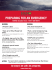### Country Club Brochure - San Joaquin County GIS Map Server Home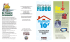### instructions - CallisonRTKL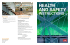### 831- 454-7303 #1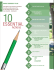### Evacuation Routes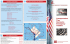### instructions - CallisonRTKL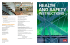### Safer School Combined Tsunami Drill in Suva